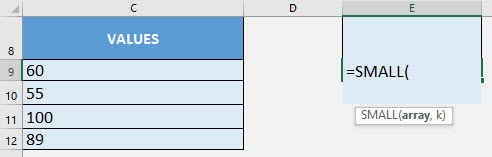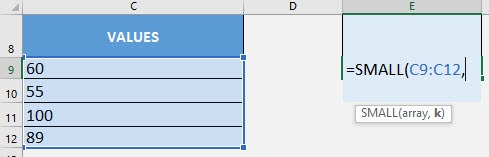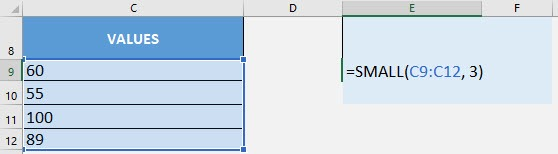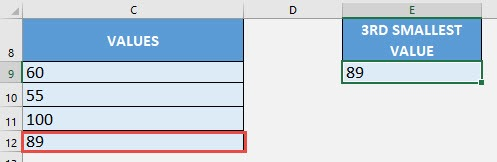What does it do?

Get the nth smallest value from a range of values

Formula breakdown:

=SMALL(array, k)

What it means:

=SMALL(range of values, position of the smallest value)

You have a list of values and you want to get the third (or nth) smallest value, no problem! Excel’s SMALL Formula can easily get that for you!

I explain how you can do this below:STEP 1: We need to enter the SMALL function in a blank cell:

## =SMALL(STEP 2: The SMALL arguments:

## array

What is the range of values?

Select the cells containing your values:

## =SMALL(C9:C12,## k

What is the nth smallest value that you want to get?

We want to get the third smallest value so we will place in 3.

## =SMALL(C9:C12, 3)You now have your third smallest value!How to Use the SMALL Formula in Excel